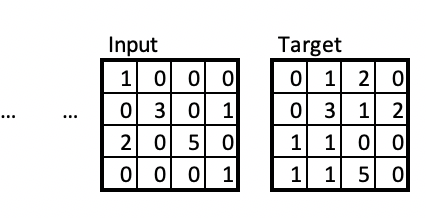# From MSE to Poisson loss - Car Demand Prediction

Hi,

I’m working on a project where we wish to predict demand for a free-floating car-sharing service using Conv-LSTM’s.

The problem is formulated as aggregate demand in grid-cells, where each grid cell is around 1 km^2 and the value contained in this cell is the number of successful rentals. Using this formulation we have a time series of 60-minute windows as our data. This gives an array of slices as input and a single slice as output - example shown belowWe have achieved mediocre performance with this setup and the use of the MSE-loss but would like to give the Poisson-loss a try.

Our problem is that we end up with a negative loss after a few iterations of training and are unsure about how to identify the problem or if there is something we are unaware of.

We use the Adam optimizer and with the following settings for the criterion:

``````criterion = nn.PoissonNLLLoss(log_input=False)
``````

Using the default log_input=True did not help.

Our traning loop looks a bit like this where HORIZONTAL_LINES and VERTICAL_LINES are related to the grid sizing.

``````model.train()
# For grid in traning set
for i, (inputs, targets) in enumerate(train_loader):
x = inputs.unsqueeze(2)
y = targets.view(1,1,1,HORIZONTAL_LINES+1,VERTICAL_LINES+1)

# Forward pass to get output/logits
y_hat = model.forward(x, future_seq=1)

loss = criterion(y,y_hat)

loss.backward()

# Updating parameters
optimizer.step()

# Update loss
epoch_training_loss += loss.cpu().detach().numpy()
``````

We were considering if our problem is because we have a very sparse dataset (average cell value is around 1) and with 0 a lot of the time, or the fact that our model predicts in the continuous range instead of discrete values.

The architecture of the model is based on the following model, but with slight such as nn.ReLU as the activation fuction.

Any help would be much appreciated

Your y,y_hat order is reversed from the correct one.